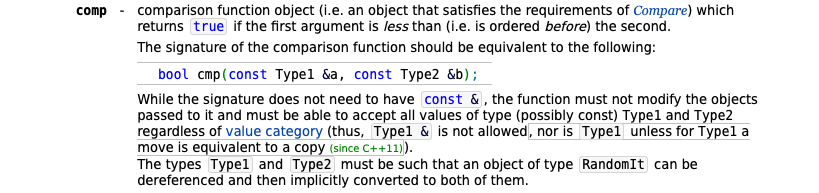# sort a vector or pair alphabetically.

• ``````#include <iostream>     // std::cout
#include <algorithm>    // std::sort
#include <vector>       // std::vector

bool compareFunction (std::string a, std::string b) {return a<b;}
//compare any way you like, here I am using the default string comparison

int main ()
{
std::string myNames[] = {"Henry","Tom","Jafar","Alice","Bob","Cindy","Clara","Michael"};

std::vector<std::string> myvector (myNames, myNames+8); //create vector from array

std::sort(myvector.begin(),myvector.end(),compareFunction);//sort the vector

std::cout << "Sorted vector:";
for (std::vector<std::string>::iterator it=myvector.begin(); it!=myvector.end(); ++it)
std::cout << ' ' << *it;
std::cout << '\n';

return 0;
}
``````

http://cpp.sh/7hrvf

how would this apply to my vector?

``````vector< pair <string, string> > data; // name / email
``````

takes 2 arguments.

``````bool compareFunction (std::string a, std::string b) {return a<b;}
``````

but i don't see these arguments applied here.

``````std::sort(myvector.begin(),myvector.end(),compareFunction);//sort the vector
``````

i need the "key" value of my pairs fully alphabetically sorted up to the last char of the name.

regards.

• @Natural_Bugger sorry for asking, but how is this issue related to Qt specifically?

It seems like an initial assignment in a programming course

• @Pablo-J-Rogina

well, I'm solving some online programming "riddles" and since i use Qt creator, because i like it.
been rusty for years.

• @Natural_Bugger

bool compareFunction (std::pair<std::string, std::string> a, std::pair<std::string, std::string> b) {return a.first<b.first;}

btw, this should, even for std::string be const references

bool compareFunction (const std::pair<std::string, std::string> &a, const std::pair<std::string, std::string> &b) {return a.first<b.first;}

• @Natural_Bugger
If you wish to store `std::pair()` elements in your vector, you need a `compareFunction()` which takes `std::pair()` instead of `std::string` as its arguments (well, const references to them really, but that's not the point).

• bool compareFunction (const std::pair<std::string, std::string> &a, const std::pair<std::string, std::string> &b) {return a.first<b.first;}

indeed, thank you very much.
i thought i had to expand the function and use some more "std::.." functions, but it worked perfectly.

but I'm still a little clueless on how the function receives it arguments.

@JonB

also to you, thnx

• but I'm still a little clueless on how the function receives it arguments.

this is taken from the documentationThe argument for the compare, expects a function that returns a bool and accepts 2 arguments

• but I'm still a little clueless on how the function receives it arguments.

When you call `std::sort()` on a vector of objects, internally it will repeatedly call the function you pass as `compareFunction` on pairs of elements in the vector as it goes along, using its return result to re-order these elements in the vector, till they are fully sorted. All you need to know is that the argument types to `compareFunction` must match exactly the type of the elements in the vector, else you will get a compile-time type error.

• thnx, i placed the sort function at the right spot in the first moment and it worked out perfectly first time.

it just wasn't obvious on how the "compareFunction" receives it's arguments.
that's clever, it wouldn't be obvious to most at first sight.

``````std::sort(myvector.begin(),myvector.end(),compareFunction);//sort the vector
``````

no arguments are passed to "compareFunction", whilst requiring 2 arguments

``````bool compareFunction (std::string a, std::string b) {return a<b;}
``````

thnx @J-Hilk and @JonB

• @Natural_Bugger
`compareFunction` is passed to `std::sort()` without any following parentheses, i.e. not `compareFunction(...)`. That means at this point is does not call the function, it merely passes the address of the function. `std::sort()` works through the vector element range you passed, taking pairs of elements and now calling `compareFunction(element1, element2)`.